output.to from Sideway
Calculus

Draft for Information Only

# Content

``` Rules of Integration for Definite Integral  Definite Integral Properties ```

# Rules of Integration for Definite Integral

In order to simplify the task of finding integrals, some general rules are developed to help finding definite integrals with having to use the definition directly.

## Definite Integral Properties

Some useful properties of definite integrals

1. Definite integral as limit of function sum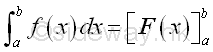Proof: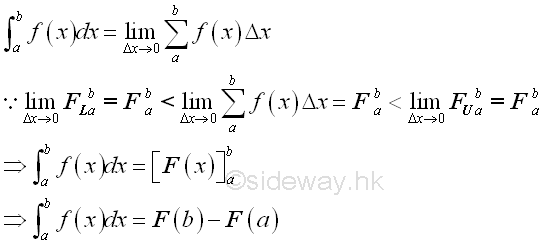2. additivity of intervals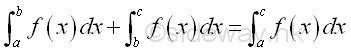Proof: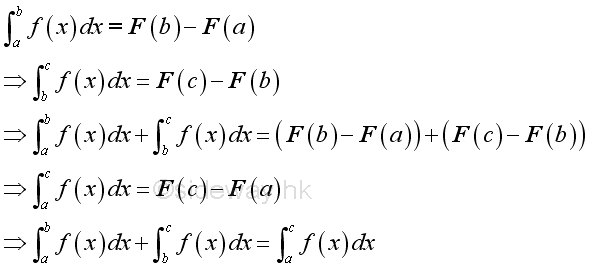3. zero length of interval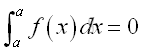Proof: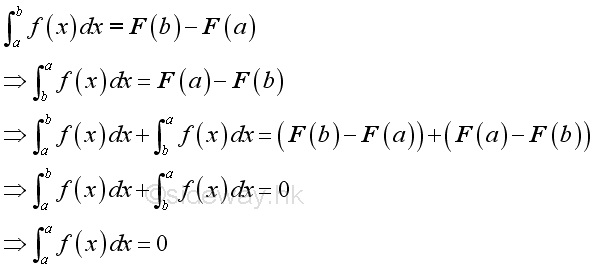4. reversing of limits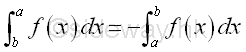Proof: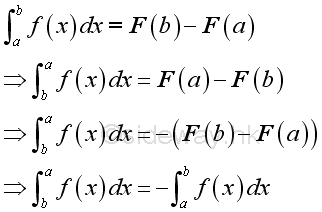5. subtraction of intervals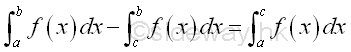Proof: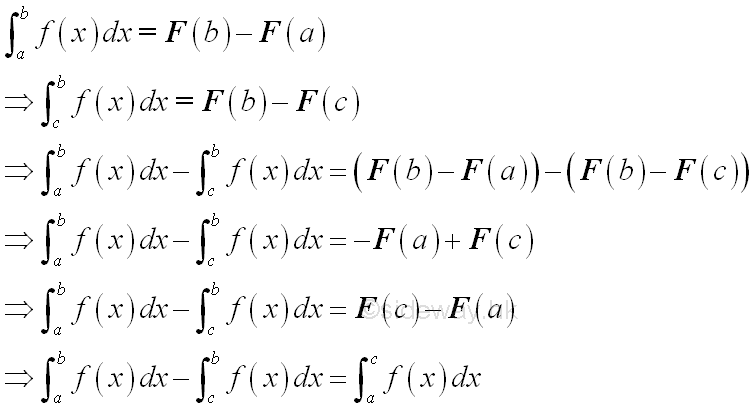6. Constant Multiple Rule of definite integral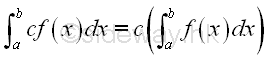Proof: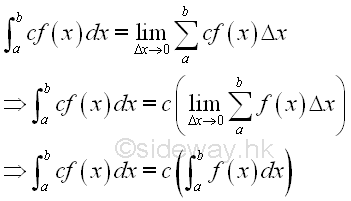7. Constant Multiple Rule of Identity function of definite integral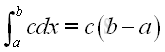Proof: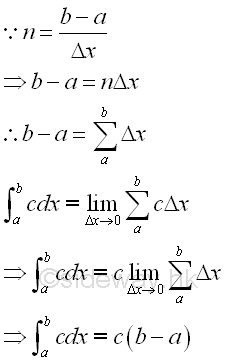8. Constant Rule of zero function of definite integral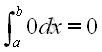Proof: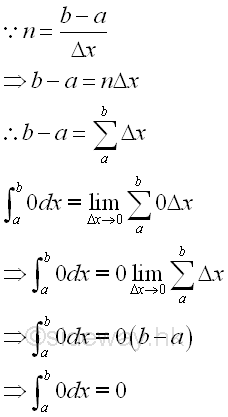9. Sum of Functions Rule of definite integral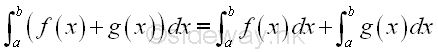Proof: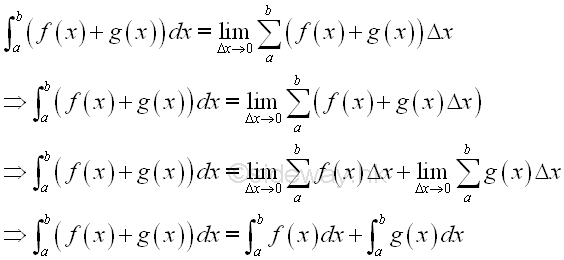10. Difference of Functions Rule of definite integral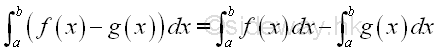Proof: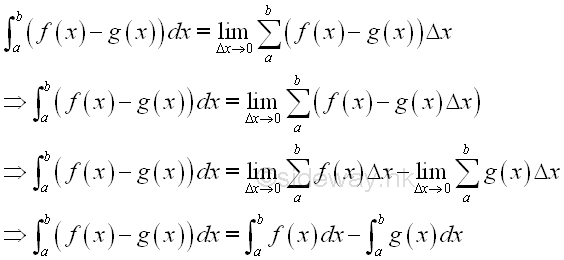©sidewayReferences

1. S. James, 1999, Calculus, Brooks/Cole Publishing Co., USA
2. B. Joseph, 1978, University Mathematics: A Textbook for Students of Science & Engineering, Blackie & Son Limited, HongKongID: 111000019 Last Updated: 10/22/2011 Revision: 1 Ref:Home (5)

Business

Management

HBR (3)

Information

Recreation

Hobbies (7)

Culture

Chinese (1097)

English (336)

Reference (66)

Computer

Hardware (149)

Software

Application (187)

Digitization (24)

Numeric (19)

Programming

Web (644)CSS (SC)

ASP.NET (SC)

HTML

Knowledge Base

Common Color (SC)

Html 401 Special (SC)

OS (389)MS Windows

Windows10 (SC)

.NET Framework (SC)

DeskTop (7)

Knowledge

Mathematics

Formulas (8)

Number Theory (206)

Algebra (20)

Trigonometry (18)

Geometry (18)

Calculus (67)

Complex Analysis (21)

Engineering

Tables (8)

Mechanical

Mechanics (1)

Rigid Bodies

Statics (92)

Dynamics (37)

Fluid (5)

Control

Acoustics (19)

Biology (1)

Geography (1)

Latest Updated Links

Copyright © 2000-2019 Sideway . All rights reserved Disclaimers last modified on 10 Feb 2019